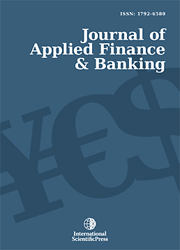# Journal of Applied Finance & Banking

#### Capital Asset Pricing Model – investigation and Testing

•[ Download ]
• Times downloaded: 957
• Abstract

This paper aims to develop testing model based on logistic regression with three factors to investigate the equity premium portion of CAPM model. It includes (1) literature review on equity premium of CAPM (Capital Asset Pricing Model) model; (2) Set up logistic regression model; (3) Data collection from Datastream; (4) Use of Matlab in regression; (5) Data input in logistic regression; (6) Create a homemade model to prove the nonexistence of equity premium puzzle. Together with investigating the proper definition of risk-free rate, this paper investigates and tests the basic model of CAPM.

JEL classification numbers: G1
Keywords: CAPM model, risk-free rate, risk premium, logistic regression, volatility index.ISSN: 1792-6599 (Online)
1792-6580 (Print)